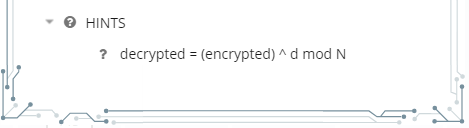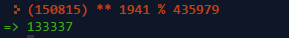# PicoCTF 2017 – computeRSA

Another day, another challenge.

Today’s blog post will explore solving the “computeRSA” challenge in the PicoCTF challenge.

Let’s get started.

Clicking on the challenge we see the following:OK we need do a calculation for the RSA algorithm. Since we have the encrypted number, then we need to do the decryption.

Let’s look at the hints.We have the decrypted formula.

So the formula: (150815) ^ 1941 mod 435979

Let’s go to Google and use a python interpreter to find the decrypted number.

Going to the following link we’re presented with the interpreter.

Entering the formula in the interpreter we get the following:Entering this number as the flag we’ve acquired 50 points!